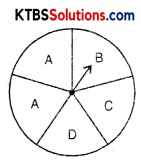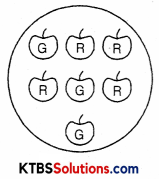# KSEEB Solutions for Class 8 Maths Chapter 5 Data Handling Ex 5.3

## KSEEB Solutions for Class 8 Maths Chapter 5 Data Handling Ex 5.3

Question 1.
List the outcomes you can see in these experiments.
(a) Spinning wheelSolution:
(a) Outcomes in the spinning wheel are A, B, C, and D.

Question 2.
When a disc is thrown, list the outcomes of an event of getting
(i) (a) a prime number
(b) not a prime number.
(ii) (a) a number greater than 5
(b) a number not greater than 5
Solution:
(i) (a) A prime number 2, 3, 5
(b) Not a prime number 4, 6

(ii) (a) A number greater than 5 = 6
(b) A number not greater than 5 = 1, 2, 3, 4Question 3.
Find the:
(a) Probability of the pointer stopping on D in [Question 1-(a)]?
(b) Probability of getting an ace from a well-shuffled deck of 52 playing cards?
(c) Probability of getting a red apple. (See figure below)Solution:
(a) Number of possible outcomes for pointer stopping on
D = 1
Total number of outcomes of the experiment = 5
∴ Probability of pointer stopping at D = $$\frac{1}{5}$$

(b) Total number of aces = 4
Total number of outcomes = 52
P(an ace) = $$\frac{4}{52}=\frac{1}{13}$$

(c) Number of Red apples = 4
Total number of outcomes = 7
∴ P of getting a red apple = $$\frac{4}{7}$$

Question 4.
Numbers 1 to 10 are written on ten separate slips (one number on one slip), kept in a box and mixed well. One slip is chosen from the box without looking into it. What is the probability of:
(i) getting a number 6?
(ii) getting a number less than 6?
(iii) getting a number less than 6?
(iv) getting a 1-digit number?
Solution:
(i) Number of slip written 6 on it = 1
Total number of outcomes = 10
∴ P (getting 6 on a slip) = $$\frac{1}{10}$$

(ii) Number less than 6 are = 1, 2, 3, 4, 5 = 5
Total number of slips = 10
∴ P(getting a number less than 6) = $$\frac{5}{10}=\frac{1}{2}$$(iii) Number greater than 6 are = 7, 8, 9, 10 = 4
Total number of slips = 10
∴ P (getting a number greater than 6) = $$\frac{4}{10}=\frac{2}{5}$$

(iv) One digit numbers are = 1, 2, 3, 4, 5, 6, 7, 8, 9
Total numbers = 10
∴ P (of one digit number) = $$\frac{9}{10}$$

Question 5.
If you have a spinning wheel with 3 green sectors, 1 blue sector, and 1 red sector, what is the probability of getting a green sector? What is the probability of getting a non-blue sector?
Solution:
Total sectors = 3 + 1 + 1 = 5
Number of green sectors = 3
Number of non-blue sectors = 3 + 1 = 4
∴ P(of getting a green sector) = $$\frac{3}{5}$$
∴ P (of getting a non blue sector) = $$\frac{4}{5}$$Question 6.
Find the probabilities of the events given in Question 2.
Solution:
(i) (a) P(a prime number) = $$\frac{3}{6}=\frac{1}{2}$$
(b) P(not a prime number) = $$\frac{2}{6}=\frac{1}{3}$$

(ii) (a) P(a number greater than 5) = $$\frac{1}{6}$$
(b) P(a number not greater than 5) = $$\frac{4}{6}=\frac{2}{3}$$